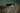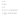Math Methods – Differentiation 3/5 – Exponential etcStep 1
>
Step 2
>
Step 3
>
Step 4
How would you like to be tutored?
Online
In Person

# Math Methods – Differentiation 3/5 – Exponential etc

Once you know basic differentiation and can use both the Product and Quotient Rules, you need to get the hang of differentiating more tricky expressions. This short course will help you do just that with tips and tricks, not to mention a few exercises to get the hang of it too! If you want to benefit from this, be sure to spend a good hour at making sure you understand every step in the worked examples. If it’s too tricky, ask a tutor or someone who knows!

Exponential

An exponential expression is when you elevate a number to the power of x, for example :

2x     ax     ex     and so on…

The derivatives are EASY, it’s just the same expression multiplied by loge of the number under the power. So for our 3 expressions in the preceding paragraph, the derivatives are :

2x loge(2)     ax loge(a)     ex loge(e) = ex

The last case is a special one because loge(e) = 1. You need to remember the very special case that the derivative of ex is ex ! It doesn’t change!

Tip: if a number is multiplying the exponential, it doesn’t influence the derivative. For example :

• The derivative of 5ex is also 5ex.
• The derivative of 3 . 2x is 3 . 2x loge(2)

Logarithms

The derivative of a log with any base “a” of x is: 1 / (x . loge(a))

In the special case where the base is “e” the derivative is simply 1 / x

Notation : Sometimes loge(x) is written ln(x) (pronounced “natural log of x”)

Roots

The derivative of roots can be found the same way as the derivative of polynomials. You simply need to transform the root into an appropriate power of x. For example :So using the usual method of differentiation for polynomials, bring the power to the front, then bring the power down by one unit. For example :

The derivative ofExercises: Find the derivatives of the following expressions.